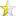• Articles
• The difference between pointers and arra
Jan 14, 2008

# The difference between pointers and arraysThe difference between pointers and arrays

I have seen in many places that an array is introduced as a pointer. This is technically not correct. Arrays are not pointer. So what is it? It is like any other variable in C++.
Take a look at this code:

 ``12`` ``````int arr={3,4,5}; cout<

You may say: "Look there, arr is an address, who says it's not a pointer?"
And I say: this code prints out an address. So var is an address?

 ``12`` ``````int var; cout<<&var;``````

Let me explain:
All the variables can be manipulated in the memory by their addresses. CPU uses these addresses to fetch them, change them and save them. So, all variables have an address (not only those that you pointed to them). We can find out the address of a variable by (&) operator, in form of:

 ``12`` ``````int* ptr=&intvar; // Now the address of intvar is in ptr ``````

An array is just a sequence of variables. But there is a rule, that C++ looks arrays as if they were pointers. What does it means? It means that if you write arr, compiler takes it as &arr (except in three conditions that I'll tell you soon), that is the address of the first element of the array. It has a type "pointer to T" where T is the type of array elements (that is int in our example). If you add 1 to it it will point to the second element of the array.

So it takes &arr as what?
It is one of the exceptions where the rule does not apply. It takes it as a pointer to array. It will again point to the first element of the array, but if you add one to this pointer, it will point to the address of the place right after the last element of the array (just like you skipped the array). It is a pointer to the whole the array. The value of &arr and arr is the same (address of the first element). But their type is different. Here &arr has type "pointer to the array of T" (compare it to the type of arr).

Look at this

 ``12345`` ``````int arr={3,4,5}; cout<<"First element of the array: "<

First cout: Prints out the "value" of arr.
Second cout: Prints out the "address" of arr.
Third cout: Again prints out the "address" of arr.
Forth cout: Prints out the address of the array, that is again the address of arr.

And this:

 ``12345`` ``````int arr={3,4,5}; cout<<"First element of the array: "<

First cout: Prints out the "value" of arr plus one.
Second cout: Prints out the "address" of arr.
Third cout: Again prints out the "address" of arr .
Forth cout: Prints out the first address of memory after the array.

Comparison
Similarities, each with an example:
1) (*) can be used for both.

 ``12345678`` ``````int arr={1,3,4}; //Declares an array with 3 elements int * ptr=arr; //Initialize pointer ptr with the address of array arr cout<<*(arr+2)<

2) Subscription can be used for both

 ``12345678`` ``````int arr={1,3,4}; //Declares an array with 3 elements int * ptr=arr; //Initialize pointer ptr with the address of array arr cout<

3) A pointer can be used as an array.
 ``1234567`` ``````int *ptr=new int; ptr=12; ptr=3; cout<

4) An array can have a type of pointer. That means the elements of it can be pointers.

 ``12345678910111213141516171819`` ``````int ar={8,2}; int var1=66; int var2=111; int* ptarray; ptarray=ar; ptarray=&ar; //Address of second element of array ar ptarray=&var1; ptarray=&var2; ptarray=&ar; // To keep code small I use a loop for(int i=0;i<5;i++) cout<<*(ptarray)<

5) All arrays will be passed to functions as a pointer, which means you can't actually send an array to a function. So function(char[]) is equal to function(char*).

 ``123456789101112131415`` ``````#include using namespace std; void test(char v[]); int main(){ char a; //a="If a is an array, this line should generate an error"; test(pta); return 0; } void test(char v[]){ v="If v is an array, this line should generate an error"; cout<

Differences:

1) A pointer is a place in memory that keeps address of another place inside, while an array is a single, preallocated chunk of contiguous elements (all of the same type), fixed in size and location.

3) Array like pointers can't be initialized at definition like arrays.

 ``12`` ``````char car={'a','b',66}; char* cpt=new char; //No way to be initialized here. ``````

4) When we allocate memory for a pointer to use it as a dynamic array. The memory can be resized or freed later. But this is not the case for arrays.

For example:

 ``123`` ``````char* pta=new char; //Using pta delete[] pta;``````

3) They produce different assembly code. Look and compare:

 ``12345678910`` ``````int main() { char arr; char* ptr=new char; arr='C'; //Assembly is for this. ptr='p'; //And for this. return 0; }``````

Will have an assembly like this:

 ``123456`` ``````arr='C'; mov byte ptr [ebp-4],43h //The code for putting one character in an array ptr='p'; mov ecx,dword ptr [ebp-8] //The two line code for putting one character mov byte ptr [ecx],70h //in a place where a pointer points to ``````

Hope to be useful...

Refferences:
http://67.40.109.61/torek/c/index.html
http://c-faq.com/questions.html